# Problems and solutions in financial management step by step approach

1 de Dec de 2013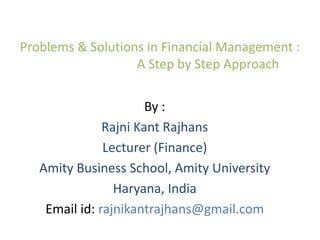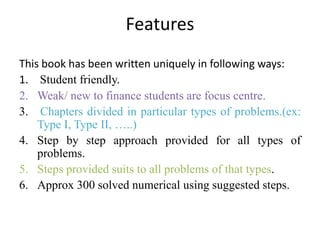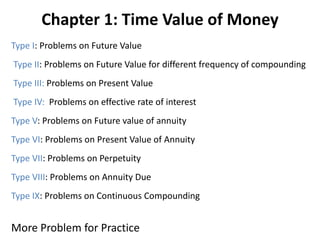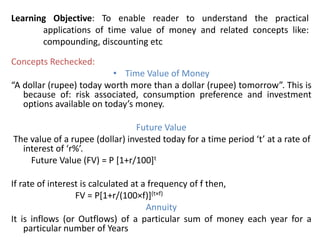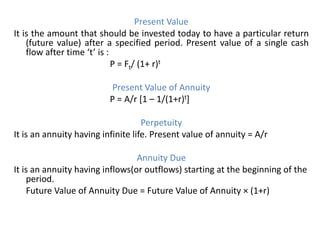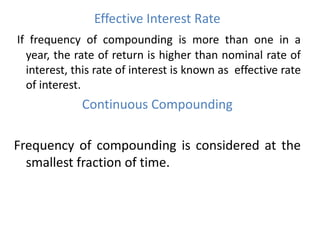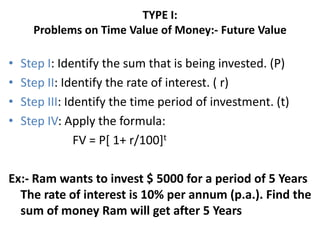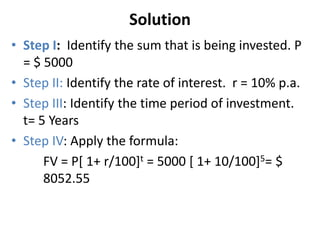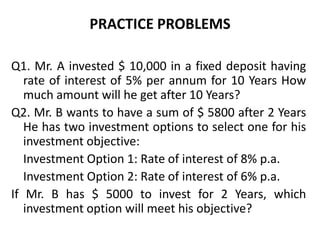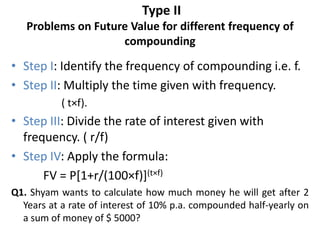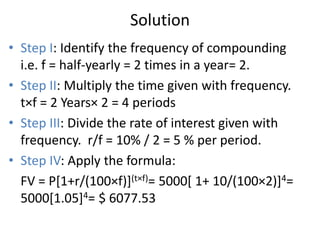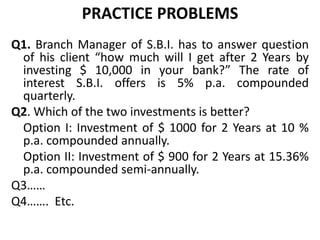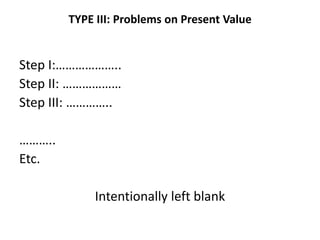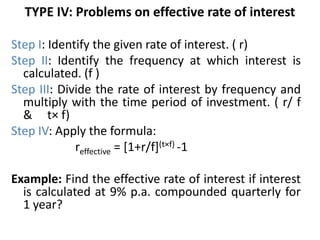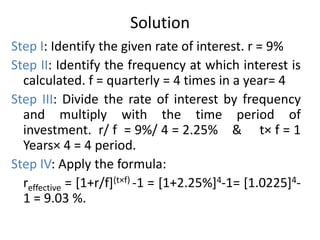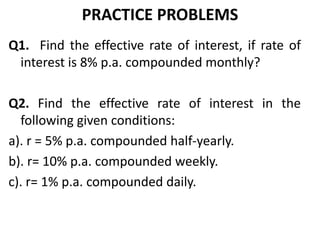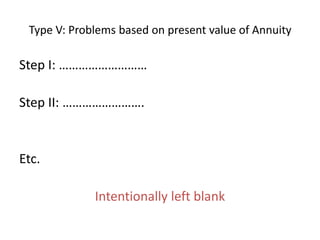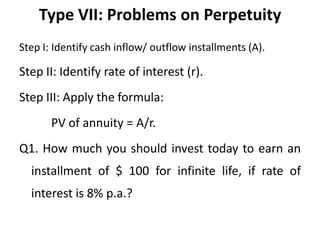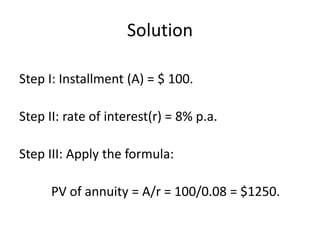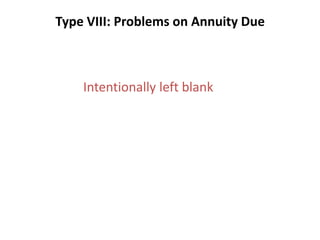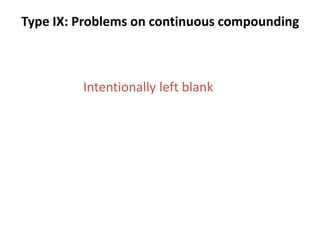1 de 22

### Problems and solutions in financial management step by step approach

• 1. Problems & Solutions in Financial Management : A Step by Step Approach By : Rajni Kant Rajhans Lecturer (Finance) Amity Business School, Amity University Haryana, India Email id: rajnikantrajhans@gmail.com
• 2. Features This book has been written uniquely in following ways: 1. Student friendly. 2. Weak/ new to finance students are focus centre. 3. Chapters divided in particular types of problems.(ex: Type I, Type II, …..) 4. Step by step approach provided for all types of problems. 5. Steps provided suits to all problems of that types. 6. Approx 300 solved numerical using suggested steps.
• 3. Chapter 1: Time Value of Money Type I: Problems on Future Value Type II: Problems on Future Value for different frequency of compounding Type III: Problems on Present Value Type IV: Problems on effective rate of interest Type V: Problems on Future value of annuity Type VI: Problems on Present Value of Annuity Type VII: Problems on Perpetuity Type VIII: Problems on Annuity Due Type IX: Problems on Continuous Compounding More Problem for Practice
• 4. Learning Objective: To enable reader to understand the practical applications of time value of money and related concepts like: compounding, discounting etc Concepts Rechecked: • Time Value of Money “A dollar (rupee) today worth more than a dollar (rupee) tomorrow”. This is because of: risk associated, consumption preference and investment options available on today’s money. Future Value The value of a rupee (dollar) invested today for a time period ‘t’ at a rate of interest of ‘r%’. Future Value (FV) = P [1+r/100]t If rate of interest is calculated at a frequency of f then, FV = P[1+r/(100×f)](t×f) Annuity It is inflows (or Outflows) of a particular sum of money each year for a particular number of Years
• 5. Present Value It is the amount that should be invested today to have a particular return (future value) after a specified period. Present value of a single cash flow after time ‘t’ is : P = Ft/ (1+ r)t Present Value of Annuity P = A/r [1 – 1/(1+r)t] Perpetuity It is an annuity having infinite life. Present value of annuity = A/r Annuity Due It is an annuity having inflows(or outflows) starting at the beginning of the period. Future Value of Annuity Due = Future Value of Annuity × (1+r)
• 6. Effective Interest Rate If frequency of compounding is more than one in a year, the rate of return is higher than nominal rate of interest, this rate of interest is known as effective rate of interest. Continuous Compounding Frequency of compounding is considered at the smallest fraction of time.
• 7. TYPE I: Problems on Time Value of Money:- Future Value • • • • Step I: Identify the sum that is being invested. (P) Step II: Identify the rate of interest. ( r) Step III: Identify the time period of investment. (t) Step IV: Apply the formula: FV = P[ 1+ r/100]t Ex:- Ram wants to invest \$ 5000 for a period of 5 Years The rate of interest is 10% per annum (p.a.). Find the sum of money Ram will get after 5 Years
• 8. Solution • Step I: Identify the sum that is being invested. P = \$ 5000 • Step II: Identify the rate of interest. r = 10% p.a. • Step III: Identify the time period of investment. t= 5 Years • Step IV: Apply the formula: FV = P[ 1+ r/100]t = 5000 [ 1+ 10/100]5= \$ 8052.55
• 9. PRACTICE PROBLEMS Q1. Mr. A invested \$ 10,000 in a fixed deposit having rate of interest of 5% per annum for 10 Years How much amount will he get after 10 Years? Q2. Mr. B wants to have a sum of \$ 5800 after 2 Years He has two investment options to select one for his investment objective: Investment Option 1: Rate of interest of 8% p.a. Investment Option 2: Rate of interest of 6% p.a. If Mr. B has \$ 5000 to invest for 2 Years, which investment option will meet his objective?
• 10. Type II Problems on Future Value for different frequency of compounding • Step I: Identify the frequency of compounding i.e. f. • Step II: Multiply the time given with frequency. ( t×f). • Step III: Divide the rate of interest given with frequency. ( r/f) • Step IV: Apply the formula: FV = P[1+r/(100×f)](t×f) Q1. Shyam wants to calculate how much money he will get after 2 Years at a rate of interest of 10% p.a. compounded half-yearly on a sum of money of \$ 5000?
• 11. Solution • Step I: Identify the frequency of compounding i.e. f = half-yearly = 2 times in a year= 2. • Step II: Multiply the time given with frequency. t×f = 2 Years× 2 = 4 periods • Step III: Divide the rate of interest given with frequency. r/f = 10% / 2 = 5 % per period. • Step IV: Apply the formula: FV = P[1+r/(100×f)](t×f)= 5000[ 1+ 10/(100×2)]4= 5000[1.05]4= \$ 6077.53
• 12. PRACTICE PROBLEMS Q1. Branch Manager of S.B.I. has to answer question of his client “how much will I get after 2 Years by investing \$ 10,000 in your bank?” The rate of interest S.B.I. offers is 5% p.a. compounded quarterly. Q2. Which of the two investments is better? Option I: Investment of \$ 1000 for 2 Years at 10 % p.a. compounded annually. Option II: Investment of \$ 900 for 2 Years at 15.36% p.a. compounded semi-annually. Q3…… Q4……. Etc.
• 13. TYPE III: Problems on Present Value Step I:……………….. Step II: ……………… Step III: ………….. ……….. Etc. Intentionally left blank
• 14. TYPE IV: Problems on effective rate of interest Step I: Identify the given rate of interest. ( r) Step II: Identify the frequency at which interest is calculated. (f ) Step III: Divide the rate of interest by frequency and multiply with the time period of investment. ( r/ f & t× f) Step IV: Apply the formula: reffective = [1+r/f](t×f) -1 Example: Find the effective rate of interest if interest is calculated at 9% p.a. compounded quarterly for 1 year?
• 15. Solution Step I: Identify the given rate of interest. r = 9% Step II: Identify the frequency at which interest is calculated. f = quarterly = 4 times in a year= 4 Step III: Divide the rate of interest by frequency and multiply with the time period of investment. r/ f = 9%/ 4 = 2.25% & t× f = 1 Years× 4 = 4 period. Step IV: Apply the formula: reffective = [1+r/f](t×f) -1 = [1+2.25%]4-1= [1.0225]41 = 9.03 %.
• 16. PRACTICE PROBLEMS Q1. Find the effective rate of interest, if rate of interest is 8% p.a. compounded monthly? Q2. Find the effective rate of interest in the following given conditions: a). r = 5% p.a. compounded half-yearly. b). r= 10% p.a. compounded weekly. c). r= 1% p.a. compounded daily.
• 17. Type V: Problems based on present value of Annuity Step I: ……………………… Step II: ……………………. Etc. Intentionally left blank
• 18. Type VII: Problems on Perpetuity Step I: Identify cash inflow/ outflow installments (A). Step II: Identify rate of interest (r). Step III: Apply the formula: PV of annuity = A/r. Q1. How much you should invest today to earn an installment of \$ 100 for infinite life, if rate of interest is 8% p.a.?
• 19. Solution Step I: Installment (A) = \$ 100. Step II: rate of interest(r) = 8% p.a. Step III: Apply the formula: PV of annuity = A/r = 100/0.08 = \$1250.
• 20. Type VIII: Problems on Annuity Due Intentionally left blank
• 21. Type IX: Problems on continuous compounding Intentionally left blank
• 22. Thank You For detailed slides of more chapter (s) contact me @ rajnikantrajhans@gmail.com